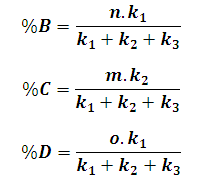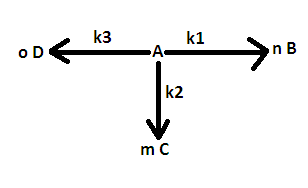# Parallel Reaction Product Percentage Calculator

This Calctown Calculator calculates the percentage of product among the products in a parallel reaction at any point of time during the course of a reaction.

s-1
s-1
s-1

#### ResultClick here to view image

where

%B, %C, %D = percentage of respective products among products

k1, k2 and k3 are the respective reaction rates

n, m and o are respective coefficient of product formation